# Space of mappings, topological

(diff) ← Older revision | Latest revision (diff) | Newer revision → (diff)

A setof mappings from a setinto a topological spacewith some natural topologyon. For fixedandone obtains different spaces of mappings, depending on which mappings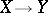are included inand what natural topologyis endowed with. The choice ofis related to the presence of additional structures onandand to peculiarities of the situation considered. Thus, forone can take: the set of all continuous mappings, the set of all mappings, the set of all continuous linear mappings from a topological vector spaceinto a topological vector space, the set of all continuous homomorphisms from a topological groupinto a topological group, the set of all smooth mappings from an interval into the straight line, etc.

The importance of considering spaces of mappings is to a certain extent related to the fact that mappings are the most general method of comparing mathematical objects.

Natural topologies (on) are usually determined in the following way. A familyof subsets ofis fixed, and a pre-base for a topologyonis formed by sets of the form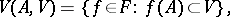where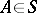andis an open set in. Ifis the family of finite (or singleton) subsets of, thenis called the topology of pointwise convergence on. Ifconsists of all compact subsets of, thenis called the compact-open topology. If, thenis called the topology of uniform convergence (on). Moreover, every topologyonobtained by this scheme is called the topology of uniform convergence on elements of.

Depending on the branch of mathematics, some spaces of mappings turn out to be especially important. Among the central objects in functional analysis one counts the Banach spaces of continuous functions on compacta in the norm topology, i.e. the topology of uniform convergence, and in the weak topology, which can be described in terms of pointwise convergence. In homotopy theory an important role is played by the path space of a topological space, i.e. the space of continuous mappings from a closed interval into this topological space. Homotopy of one mapping into another is represented by a path in the space of mappings. The space of mappings from a sphere into a sphere arises in the definition of homotopy and cohomotopy groups.

The compact-open topology on the set of mappings of one-space into another turns out to be especially natural. An advantage of the topology of uniform convergence (on the entire space) is its metrizability. This topology is the strongest in a large class of natural topologies on a space of mappings. However, the topology of pointwise convergence too has its advantages — as the weakest in this class of topologies. First, this topology best reflects the compactness, and compactness is one of the most useful properties of a set of functions. Secondly, there is the fundamental result of J. Nagata that puts the study of arbitrary Tikhonov spaces (cf. Tikhonov space) in direct relation to the study of topological rings. More precisely, two Tikhonov spaces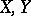are homeomorphic if and only if the topological rings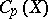and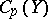of continuous functions onand, respectively, with the topology of pointwise convergence are topologically isomorphic.

The consideration of topological properties of spaces of mappings is useful in proving theorems on the existence of mappings with some property. The completeness of the metric space of continuous real-valued functions on a compactum is used, via the contraction-mapping principle, in the proof of the fundamental theorem on the existence of a solution to a differential equation under certain assumptions. A consequence of the completeness of a metric space of functions is the Baire property. Using it one can prove, e.g., the existence of a continuous nowhere-differentiable function on an interval. The Baire property of spaces of functions plays a central role in the proof of the theorem on general position, in the proof of the well-known theorem on the imbeddability of each-dimensional compactum with a countable base into-dimensional Euclidean space, etc.

The influence of spaces of real-valued functions on general topology becomes clear in the following problem, of a general character: In what way are the properties of two spacesandrelated if the spaces of continuous real-valued functions on them (in the topology of pointwise convergence, in the compact-open topology) are homeomorphic (linearly homeomorphic). It is known, e.g., that linear homeomorphisms preserve compactness and dimension.

The fact that duality between properties of a topological space and topological properties of the space of functions on them with the topology of pointwise convergence is inherited is of special significance. As an example of a useful result in this respect one can invoke the following theorem: Any finite power of a space is Lindelöf if and only if the space of functions on it has countable tightness. This result is used, in particular, in the study of the structure of Eberlein compacta — compacta in Banach spaces, endowed with the weak topology.

How to Cite This Entry:
Space of mappings, topological. Encyclopedia of Mathematics. URL: http://encyclopediaofmath.org/index.php?title=Space_of_mappings,_topological&oldid=11579
This article was adapted from an original article by A.V. Arkhangel'skii (originator), which appeared in Encyclopedia of Mathematics - ISBN 1402006098. See original article